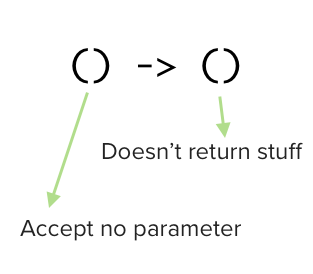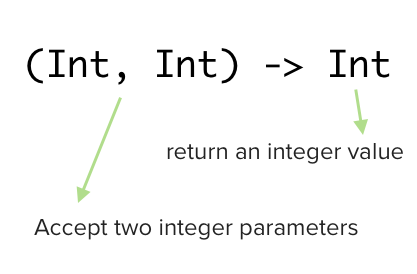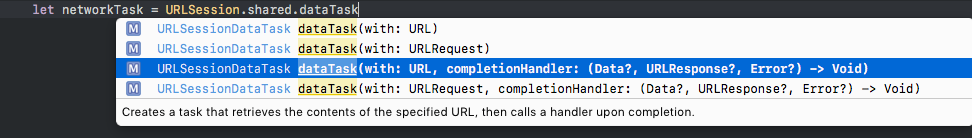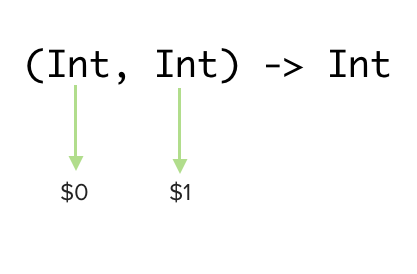Closures are very common in Swift, you might have used it before on Alamofire / URLSession call like this :

``````// {response in ... } is the closure
Alamofire.request("https://httpbin.org/get?users").validate().responseJSON { response in
// parse the json from response here
}
``````

or at the completion handler of `present` method in a view controller :

``````let storyboard = UIStoryboard(name: "Main", bundle: nil)
let settingsVC = storyboard.instantiateViewController(withIdentifier: "settingsVC")

// the completion parameter accept a closure
present(settingsVC , animated: true, completion: {
print("⚡️this will show after the settings view controller is presented on screen")
})
``````

In this post, we will give an overview about what is closure and how to use it.
Prerequisite: This post assume you already know what is a function.

## What is a closure?

Apple official documentation define closure as follows:

Closures take one of three forms:

1. Global functions are closures that have a name and do not capture any values.

2. Nested functions are closures that have a name and can capture values from their enclosing function.

3. Closure expressions are unnamed closures written in a lightweight syntax that can capture values from their surrounding context.

Global function is the usual function you define :

``````func printFoxes(){
print("🦊🦊🦊")
}
``````

Nested function means function inside a function :

``````func printAnimal(){
// this is the nested function
func printFoxes(){
print("🦊🦊🦊")
}

printFoxes()
}
``````

Closure expression can be something like this :

``````var closure: (Int, Int) -> Int = { (number1, number2) in
return number1 + number2
}

closure(2,3)
// return 5
``````

If you have used functions before, you have already used a closure. The purpose of this section is to show that function and closure expression are very identical.

I know, I know, the stuff you are curious about is the closure expression, we will further explain it in the next section.

## What is a closure expression?

Say normally we write a function like this :

``````func printFoxes(){
print("🦊🦊🦊")
}

printFoxes()
// console will output 🦊🦊🦊
``````

We can turn it into a closure expression like this :

``````// remove 'func printFoxes()', and assign it to a variable
var printFoxesClosure = { print("🦊🦊🦊") }

printFoxesClosure()
// console will output 🦊🦊🦊
``````

`{ print("🦊🦊🦊") }` is the closure expression, which is enclosed between `{` and `}`, then we assign it to a variable printFoxesClosure. To execute the code between `{` and `}` , you add parenthese `()` after the variable name like this `printFoxesClosure()`. "Wait.. this sounds like function.." exactly! Remember earlier we mentioned that function is a type of closure? Closure expression behaves like a function too.

For a function that takes parameter :

``````func addNumber(number1:Int, number2:Int) -> Int{
return number1 + number2
}

// return 7
``````

We can turn it into a closure like this :

``````// remove 'func addNumber'
// move the '(number1: Int, number2: Int) -> Int' to inside '{'
// add 'in' before writing the code to be executed inside the closure
// assign it to a variable
var addNumberClosure = {(number1: Int, number2: Int) -> Int in
return number1 + number2
}

// return 7
``````

As you have guessed it, `(number1: Int, number2: Int) -> Int` at the start of the closure is for accepting parameter and return type, same like a normal function. The `in` keyword is used to separate the input parameters / return type and the code inside the closure.

Similar to function, the syntax for declaring a closure is like this :
{`(parameter)` -> `return type` in
`your code here`
}

If the closure doesn't return, the `-> return type` can be removed :
{`(parameter)` in
`your code here`
}

If the closure doesn't have parameters and doesn't return, the `(parameter) in` can be removed as well, as there is no need to put `in` to separate input parameters/return type with the code since there is no input parameters/return type :
{
`your code here`
}

In short , closure expression is just like a function, except it doesn't have the `func` keyword and the function name.

## Explicit types of closure expression

In Swift, we can define variable like this :

``````var goodNumber : Int = 1337
var goodString : String = "The quick brown fox jumps over the lazy dog"
``````

In the above example, we explictly tell the compiler that the variable `goodNumber` is an Int (Integer) type. This is called explicit type.

Swift Compiler is quite smart as it can deduce the type of a variable by observing the value we put into the variable. Hence we can simplify the above example to this :

``````var goodNumber = 1337
var goodString = "The quick brown fox jumps over the lazy dog"
``````

The compiler is smart enough to deduce that `1337` is an Integer and `The quick brown fox jumps over the lazy dog` is a String, hence we can omit the `: Int` and `: String` in the variable declaration. This is called inferred type (variable type is inferred by the value we put in).

In the previous sections, we have used inferred type for the declaration of closure :

``````var printFoxesClosure = { print("🦊🦊🦊") }

var addNumberClosure = {(number1: Int, number2: Int) -> Int in
return number1 + number2
}
``````

Notice that there is no `: type` after the variable name in the code above. To make it explicit typed, we can define them like this :

``````var printFoxesClosure : () -> () = { print("🦊🦊🦊") }

var addNumberClosure : (Int, Int) -> Int = {(number1: Int, number2: Int) -> Int in
return number1 + number2
}
``````

What does the type `() -> ()` mean? Remember how a function is defined? eg:

``````// notice the '() -> Int' part
func testFunction() -> Int {
}
``````

Notice the similarity between `() -> ()` and the `() -> Int` in the function declaration code above? `() -> ()` means the variable type is a function, yes, the variable type is a function. This might be mindblowing for some (it did mindblow mine when I first learned about this), you can actually store a function inside a variable / constant. In fact, we have been doing this in previous sections.

In this example :

``````var printFoxesClosure : () -> () = { print("🦊🦊🦊") }

var addNumberClosure : (Int, Int) -> Int = {(number1: Int, number2: Int) -> Int in
return number1 + number2
}
``````

printFoxesClosure variable type is a function, and the function accepts no parameter and return nothing (void) .addNumberClosure variable type is a function, and the function accepts two Integer parameters and return an Integer.If you see a variable type which contain `->` in the middle, it means that variable type is a function.

If you have explicitly define the type of a closure variable / constant, you can omit the parameter type in the closure expression like this :

``````// notice that we have removed the ': Int' after number1 and number2
// and we also removed the '-> Int' before 'in'
// this is because the compiler already know that the function will take two integer and return an integer
// based on the explicit type ': (Int, Int) -> Int' we put behind the variable name
var addNumberClosure : (Int, Int) -> Int = {number1, number2 in
return number1 + number2
}
``````

## Using closure in function parameters

Closure can be used on function parameters as well. As example, one of the dataTask function from URLSession class accept a closure / function for its completionHandler parameter :The type for completionHandler is `(Data?, URLResponse?, Error?) -> Void` , means it accept a variable which its type is function, and the function accept `Data?` , `URLResponse?`, `Error` as parameters and return nothing (void) .

We can create a closure variable and put it into the completionHandler parameter :

``````// no need to define parameter type again inside the closure parameter list
// since we already inform the compiler the type of the function explicitly
// (Data?, URLResponse?, Error?) -> Void
// compiler will know data is Data? type, response is URLResponse? type and error is Error? type
// according to order
let dataTaskClosure : (Data?, URLResponse?, Error?) -> Void = { data, response, error in
guard error == nil else {
print ("error: \(error!)")
return
}

guard let content = data,
let json = (try? JSONSerialization.jsonObject(with: content, options: JSONSerialization.ReadingOptions.mutableContainers)) as? [String: Any] else {
print("Not containing JSON")
return
}
}

``````

Other than that, we can also directly put the closure in the dataTask's completionHandler parameter :

``````// similar to above, since completionHandler parameter expects (Data?, URLResponse?, Error?) -> Void type
// we can omit the parameter type in the closure, compiler will assume data has Data? type, response has URLResponse? type and error has Error? type
guard error == nil else {
print ("error: \(error!)")
return
}

guard let content = data,
let json = (try? JSONSerialization.jsonObject(with: content, options: JSONSerialization.ReadingOptions.mutableContainers)) as? [String: Any] else {
print("Not containing JSON")
return
}
})
``````

Swift has a handy shortcut, if a function's last (the rightmost) parameter type is a function / closure, we can omit the name of the last parameter and put the closure block directly after the function like this :

``````// notice we have removed 'completionHandler' parameter name
// and placed the closure directly after the function ends: (with: url){...}
guard error == nil else {
print ("error: \(error!)")
return
}

guard let content = data,
let json = (try? JSONSerialization.jsonObject(with: content, options: JSONSerialization.ReadingOptions.mutableContainers)) as? [String: Any] else {
print("Not containing JSON")
return
}
})
``````

This is known as trailing closure syntax.

## Closure shorthand argument

Ever see `\$0` or `\$1` in some Swift code and wondering what they are?
Previously we have defined addNumberClosure closure like this :

``````var addNumberClosure : (Int, Int) -> Int = {(number1: Int, number2: Int) -> Int in
return number1 + number2
}
``````

We have defined the parameters name as number1 and number2, then we referenced them in the code to add them up.

To shorten the closure, we can omit the parameters and return type as long as we have set the variable type explicitly :

``````// notice that the parameters and return type is removed in the closure
var addNumberClosure : (Int, Int) -> Int = {
// how do we access the parameters here to add them up?
}
``````

We can access the parameters using the order they are defined, `\$0` means the first parameter (from left) , `\$1` means the second parameter (from left), `\$2` means the third parameter and so on.

For the addNumberClosure, its like this :We can access the parameter like this :

``````// notice that the parameters and return type is removed in the closure
var addNumberClosure : (Int, Int) -> Int = {
// add the first parameter and second parameter
return \$0 + \$1
}
``````

This is commonly called as shorthand closure syntax / argument.

In this post I didn't explain what does `capture` and `store reference to any constants and variables in which they are defined` means as it will take another separate long post to explain these two terms. I recommend reading Closure Capture Semantincs by AliSoftware if you are interested on how capture / reference work for closure.
Closure expression is pretty much just like a function, just that it doesn't have a `func` keyword and function name. Think of closure as a portable function where you can plug it into a parameter conveniently.